I. Introduction

A. A Brief Overview

II. Stemm Theory

A. The Stemm Defined

B. c vs. The Speed of Light and the Continuum

C. Wave/Particle Duality

D. The Invariance of c and Multiple Universes

E. Expansion of the Universe and How Stemms Work

F. Propagation of Stemm States

G. Halos, Metas, Compounds, and Gravity

H. Acceleration Effect vs. Affect

I. Wave Effects and Off Sequence Stemms

J. vStemm State Interactions

K. Dark Matter and Dark Energy

L. Higgs and Other Particles

M. Anti-Matter

N. Suprasegmental Stemm Properties

O. Time in Stemm Theory

1. Special Considerations

2. Uncertainty and Relevance

III. The Way Forward

IV. Appendix

A. Rules

B. Solutions

# I. Introduction

## A. A Brief Overview

Stemm Theory assembles much of the observed phenomena in the universe, including Gravity, Dark Energy, Dark Matter, Mass/Energy and Matter, the Unidirectionality of time, multiple universes and the fabric of spacetime itself into a single unified theory.

At its most basic, Stemm Theory posits that Mass, Energy, and everything in the universe, even the fabric of the universe itself is composed of one single kind of entity, which differs only in the amount that it is moving.

In addition, Stemm Theory makes the following high level assertions:

1.        Strict Mass-Energy equivalence and intrinsic nature: There is no physical difference between Mass and Energy. They are manifestations of the same thing. The basic units in Stemm Theory do not have mass or energy, they are mass and/or energy, depending on the reference frame.

2.        Homogenous universe: the universe and everything within it is comprised of one thing, which is distributed evenly. There is no distinction between the “fabric” of the universe and the “matter” and “energy” that reside within it. It is all the same.

3.        Dark Matter and Dark Energy are undetectable because their size less than the Planck Length, however they are detectable indirectly as their mass/energy becomes increasingly evident through their effects in mass quantities.

4.        There are no extra, hidden dimensions. There are three, plus time, which is simply a byproduct of having having to describe two non-simultaneous events, which itself is necessary given that c is finite.

In many ways, Stemm Theory is similar to classical Aether Theories in positing that there is no such thing as ‘empty space’, however Stemm Theory does not posit a separate medium. Stemm theory does accommodate a pervasive simultaneity, where one basic unit can have two or more possible measurements.

Stemm Theory also echoes tenets of String Theory, in that the basic constituents of matter are constantly moving, but differs in the nature of and number of dimensions of the movement.

# II. Stemm Theory

## A. The Stemm Defined

The fabric of spacetime itself is the only material from which everything in the universe is made, the fundamental unit of which is called a Stemm (Space, Time, Energy, Mass, Movement). Stemms can be empty space, matter (energy or mass), depending on the ratio of their movement in the form of a vibration. This vibration is comprised of an expansion and contraction, the proportions of which determine their general properties that can be described using Standard Model Physics.

Although often referred to as particles, Stemms are not discrete units, but are definable regions of the fabric of spacetime.

## B. c vs. The Speed of Light and the Continuum

The value of c is generally considered to be 299,792,458 meters per second. However, the meter is defined as: "the length of the path travelled by light in vacuum during a time interval of 1/299,792,458 of a second.”

Because of the way that the speed of light has been defined, based on a distance that is a fraction a second (in order to roughly match the pre-existing length of a meter) its representation in the metric system is a whole number by design, which Stemm Theory holds to be a fundamentally flawed concept.

In Stemm Theory, c is treated as an irrational number, like, in that it cannot be quantified exactly, but can only be narrowed down with greater and greater precision. The velocity of a photon and all known particles do not equal c because their velocities can be quantified exactly.

To further explain why the speed of a photon is not equal to c, some conclusions can be drawn from Einstein’s equation, e = mc2, meaning that energy (e) is equal to mass (m) multiplied by c squared.

Rearranging the equation gives a definition of mass as well, which is m = e / c2 , or mass is energy divided by c squared. To define c, rearrange the equation to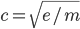.

RULE B1: As c is a velocity, Stemm Theory interprets this to mean that the only difference between Mass and Energy is velocity. In order to express this, Mass and Energy are placed at two ends of a continuum where energy increases with velocity.

This is in direct opposition to the concept of ‘relativistic mass’ in which mass increases with velocity. The Stemm Theory continuum inherently precludes any such change, and instead mass and energy are treated as two different manifestations of the same thing. However, as discussed later in section G. Stem Halos, MetaStemms and Gravity, mass can appear to increase in some situations. Ultimately more work must be done to define exactly how mass behaves under velocity.

As relativity and movement must be considered when describing mass or energy, the concept of a mass/energy continuum can be refined to incorporate the relativistic effects with a graph representing the Lorentz Factor as a function of velocity:Fig. B1: The Lorentz Factor as a function of velocity illustrates the mass-energy continuum. This graph was generated online using the function: 1/sqrt( 1 - ( (299792458*(x/10))^2 / 299792458^2) )

The Lorentz factor (the left side of Figure B1: gamma or ɣ) increases infinitely as velocity reaches c. In general relativity, this graph shows how a mass, accelerated to c, becomes exponentially more ‘massive’, thus requiring exponentially more ‘energy’ to reach c. In theory, it would be impossible to move any mass to c, since it would require infinite energy.

Since velocity can only be measured as a difference between two objects, Stemm Theory accommodates this possibility by interpreting a shift in perception along the continuum, whereby mass becomes energy as its observed velocity increases.

As the photon is not prohibited from having mass, and its upper limits have been narrowed down, Stemm Theory requires its velocity (and that of all observable particles) to be less than c.

## C. Wave/Particle Duality

Since all Stemms exist on a continuum, then where ɣ is less than infinity but greater than 1, Stemms have a nonzero percentage of both particle (mass end of the continuum) and wave (energy end) properties. Where ɣ = 1, Stemms would have no wave properties, and where ɣ = , no particle properties.

How wave properties arise from stemms will be discussed in section I. Wave Effects and Off Sequence Stemms.

The continuum also represents size and the potential for simultaneity, among other things, all of which are interlinked to velocity. These properties do not change per se, but the perception of them does from a relativistic point of view.

## D. The Invariance of cand Multiple Universes

As the photon does not travel at c, but rather c - n, then n must be an incredibly small number, so small that c-n is indiscernible from c. Since mass and energy are intrinsically linked to velocity in Stemm theory, then all Stemms that are directly perceptible (traditionally called 'matter') must have a non-zero mass.

Currently, the mass of a photon has been proven to be no more than (). On the  Stemm Theory continuum, this mass must fall within the measurement uncertainty of c: 4x10-9  or +/-0.000000004, giving a photon a speed of at least greater than 299792457.999999996 m/s.

For example, if the photon is assigned a theoretical velocity of 299792457.999999997 m/s, then its Lorentz Factor () would be ɣ  = 223529438.

With this number, a relative differential based on the Lorentz Factor that would separate a stationary object (ɣ  = 1) and a photon (ɣ  = 223529438) would be 223529437. This same difference from 223529438 to 447058876 (via), would only require an increase of 0.00000000149999999957758 m/s from the perspective of the observer at ɣ  = 1. However, from the perspective of the objects traveling at ɣ = 223529438 and 447058876, the speed between the two is perceived as 299792457.999999997 m/s.

RULE D1: Because of this relation to the Lorentz Factor, even seemingly insignificant changes in velocity are extremely important, especially when they are very high.

If ɣ is infinite when velocity equals c, then there are infinite spaces where a differential of 223529438 can occur. 223529438 and 447058876, 10000223529438 and 10000447058876 and and on and on to infinity, while still maintaining an ‘invariant’ value for c. This is a way of interpreting that c behaves as an irrational number.

RULE D2: Because of this asymptotic nature of c in relation to the Lorentz Factor, there are an infinite number of "universes" that have their own relative differentials between an observer object, and one that is traveling at c, which are parallel to this universe, using the same 'material' (Stemms), but are completely beyond perception from our frame of reference.

## E. Expansion of the Universe and How Stemms Work

The mechanism behind the expansion of the Universe is integral to Stemm Theory, as all Stemms are equivalent in their make-up, but only differ in degree of movement.

The universe is expanding not as a single entity, but is composed of an infinite number of Stemms that are expanding more or less simultaneously. Moreover, the expansion is comprised of an expansion phase, followed by a contraction phase that does not return to the original pre-expansion size, resulting in an net expansion. It is this rhythmic process that defines discrete regions perceived as particles of mass and energy, as well as space and everything in the universe.

As these expansions and contractions are imperceptible, it must be argued that at least two consecutive expansion phases happen in less than Planck Time (tP). The speed at which these expansion/contraction phases happens is irrelevant, since 1) it happens collectively on infinitely small scales, and 2) it does not transmit information, as Stemms cannot be defined on expansion or contraction alone, but on the difference between the two.

This expansion/contraction phase can be expressed as a ratio, for example, a 2:1 ratio would mean expand by two units, then contract by one, giving a net expansion of 1. A 5:2 ratio would mean expand by five units, then contract by two, giving a net expansion of 3.

The absolute net expansion distance (X), is computed by subtracting the contraction distance (Xc) from the expansion(Xe): X = Xe - Xc. The ratio of the same stemm (XR) can be given in a relative form of 1:1 to 1:0, or as an absolute value of 0.5 to 1 via.

It is important to think in these ratios, as values of X need not be defined absolutely, but rather, some general assumptions can be made regarding how they relate to each other and to the Planck Length (ℓP), which is the minimum distance that can theoretically be measured.

RULE E1: Since the universe is expanding, then for the average of all Stemms, contraction must be less than expansion, Xc < Xe.

RULE E2: For a stemm to be perceptible, such as photons, neutrinos, and other constituents considered to be matter, X must at some point exceed or meet the Planck Length, Xe >= ℓP.

Based on Rule E2, there are two main divisions between Stemms (Illustrated in figure 1):

iStemms: where expansion is less than the planck length. Xe < ℓP.

vStemms: where the Planck Length is met or exceeded at some point: Xe >= ℓP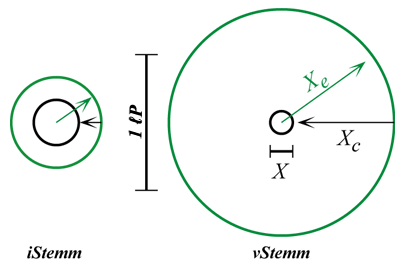Fig E1: a theoretical iStemm and a vStemm compared to the Planck Length (ℓP). The vStemm expands past the Planck Length, and is thus visible. The iStemm never exceeds the Planck Length.

Another two cases can be identified: Xe = Xc, where the contraction cancels out the expansion (XR = 1:1, ɣ = 1), and Xc = 0 where there is no contraction (XR = 1:0, ɣ = ).

In order to accommodate that the universe is expanding fastest in “empty space”, where ɣ is closer to infinity (ɣ = ∞, Xc = 0, 1:0), and slower (in and around mass) where ɣ is closer to 1 (ɣ = 1, Xc = Xe, 1:1), Stemm Theory assigns this ‘empty space’ to the far ‘energy’ end of the continuum. It is most energetic, expands fastest, and as its observed size would be less than the planck length. Its movement is imperceptible and capable of simultaneity 100% of the time, thus allowing other Stemm states to exist in the same position.

To further define Stemm properties, some other rules must be established.

RULE E3: The apparent, measurable size of all Stemms is equal to Xe, not X, which for vStemms is greater than the Planck Length.

RULE E4: As net expansion of any Stemm (Such as the observed metric expansion of space) can only be measured over great distances or time, then no Stemm has an X value greater than the Planck Length.

RULE E5: Stemms can be plotted along a Main Sequence where the expansion size and the ratio are inversely proportional to each other.

As Xe increases, XR and X decrease.For example, given a vStemm with a ratio of 1000:900 (0.52), and an iStemm of 10:2 (0.83), an intermediate Stemm that has ratio of 100:4 (0.96) or 100:95 (0.51) would be Off Sequence, and have special properties, which will be dealt with later in I. Wave Effects and Off Sequence Stemms.

RULE E6: after the contraction phase is complete, the initial Stemm has now expanded to a greater size, but maintains the same ratio value XR.

Consider an example iStemm with a ratio of 15.7:0, a high energy vStemm with a ratio of 16:0.67, and a high mass vStemm with a ratio of 50:48. In this example, the Planck Length would be between 15.7 and 16 units.

 XR start 1st cycle 1st cycle 2nd cycle 2nd cycle 3rd cycle 3rd cycle expand contract expand contract expand contract super vStemm 50:48     (0.51) 0 50 2 102 6 306 18 vStemm 16:0.67  (0.98) 0 16 15.33 261 251 4267 4099 'empty space' iStemm 15.7:0    (1) 0 15.7 15.7 262 262 4375 4375 % size of vStemm ÷ iStemm 97.64% 95.8% 93.69%

Table E1: Stemm State expansion comparison. 1st expansion = Xe , 1st contraction = Xe - Xc , 2nd expansion = (c1 × Xe) + (c1) , 2nd contraction = e2 - (Xc × c1), etc. For simplicity, only one dimension is used to compute values, actual expansion/contraction would happen in all three dimensions.

After just three cycles, both the iStemm and the vStemm have expanded dramatically compared to the much greater sized (but lower ratio) super vStemm. However, as can be seen in the percentage of size of the vStemm to the iStemm, their relative sizes are quickly separating. This is indicative of the very early state of the Universe, where all matter had yet to de-homogenize. Over cosmological time scales, the percentage will continue to drop towards zero, further shrinking vStemms compared to iStemms.

RULE E7: Over time, the expansion of all other Stemms approaches 0 compared to the expansion of 1:0 iStemms. This is consistent with traditional approaches to the expansion of the universe where empty space expands, causing all matter to become further and further apart.

## F. Propagation of Stemm States

As Stemm Theory requires Stemms to exist on the Mass/Energy Continuum, Energy is inherently tied to velocity and size and vice versa. In contrast to the Standard Model of physics, less importance is given to Potential vs. Kinetic energy, in that all Stemms have an inherent velocity. This is reinforced by the fact that particles such as Photons cannot be stopped, anymore than a Planet can be made to accelerate to c. This does not mean that a photon can’t slow down, or a planet can’t speed up if knocked out of orbit, but that these changes in velocity require changes to the component Stemms as discussed in I. Wave Effects and Off Sequence Stemms.

Matter does not move throughout the universe as discrete particles in Stemm Theory. Rather, the Stemm's Ratio (defined by XR) transfers from one Stemm (a definable region of spacetime) to the next. In this context, the ratio XR is referred to as a Stemm State.

RULE F1: More than one Stemm State can occupy the same Stemm. As the XR ratio of a Stemm State decreases from 1:0, its ability to be simultaneous with another Stemm State rapidly decreases from 100%. This means that only the most energetic, high ratio stemms have a good probability to be simultaneous.

To explain how Stemm States propagate from one Stemm to another to generate movement, a few more observations must be made:

If the propagation velocity of Main Sequence Stemm States are limited to the distance and time it takes for the Expansion (Xe, the observed “size”) to occur, then high energy vStemms would travel less distance than a higher mass vStemm.

However, if a Stemm is said to propagate at the speed and time it takes to reach size X, after the Contraction, then very high energy vStemms would travel faster than high mass vStemms as expected, but slower than 1:0 Stemms, which should be equivalent to the expansion of SpaceTime. Therefore Stemm propagation must be governed by some other mechanism.

RULE F2: The Hubble Constant describes the rate at which the Universe is expanding. H0 = 73.8 ± 2.4 (km/s)/Mpc. Calculating it down to local measurements gives 0.0000000000738 meters per second, which is about 18.6 orders of magnitude smaller than c. c can thus be stated as a velocity relative to the expansion of spacetime (1:0 Stemms):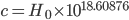As the speed of elementary particles is observationally indistinct from c, then:

RULE F3: All sub-atomic particles are composed of vStemms that have XR values very near 1:0, and that Stemms States close to 1:1 can only arise when vStemms interact to form larger vStemms (See Section G. Stemm Halos, Metas, Compounds, and Gravity).

RULE F4: By positing that vStemm states propagate at speeds as a percentage of the relation to the Hubble Constant, then for a theoretical vStemm that travels at c, its velocity must be 18.60878 orders of magnitude more than the expansion rate of a 1:0 Stemm.

The simplest way to get this size difference is to use the tentative Exponent Solution to calculate the relative velocity (vR) by solving for the X value of the vStemm (Xv) to the power of the X value of the iStemm (Xi):Following the example stemms set forth in Table E1, an ‘empty space’ iStemm has a ratio of 15.7:0 (X = 15.7, XR = 1:0). In order to find the X value of the Photon vStemm that would generate a relative propagation speed 18.6 orders of magnitude greater (i.e., propagating at c), solve for Xv via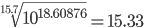.  Thus, in this example the vStemm X value must equal 15.33.  Following the Main Sequence rule (Rule E5), that XR decreases as Xe increases (), the vStemm is assigned a theoretical ratio of 16:0.67. ( X = 15.33. meaning that the Planck Length would have to be somewhere between 15.7 and 16)

From here, the appropriate propagation rate can be verified as the X value of the vStemm to the power of the X value of the iStemm, or.

Less energetic Stemms would have lower speeds, and traversing through lower energy iStemms would also decrease speed.

However, not all Stemms must stay on the Main Sequence, and in Relativistic frames, such as observing an accelerating a spaceship, or a photon being stretched by the expansion of space-time, Rule E5 can be violated.

RULE F5: If the Exponent Solution holds, then the limit of c is governed by the maximum allowable propagation rate attainable through X = 1:0 Stemms, which is broadly related to the Hubble Constant through Rules F2 and F4.

## G. Halos, Metas, Compounds, and Gravity

The “default” ratio of all stemms in the universe is 1:0. However, any stemm with a lower ratio affects the stemms around it by essentially “slowing” them down (reducing their ratio). This is called a Stemm Halo, and it extends outward in 3 dimensions.

Parent Stemm values will be notated with a suffixed with a subscript p (e.g. Xpe, XRp), and their Halo Stemms will be suffixed with h (e.g. Xhc, XRh), optionally adding a number to denote distance from the Parent. (e.g. Xh2e)

The relationship of Stemm to Stemm Halo can be broadly defined with some limits:

RULE G1: because of the lack of direct observation, Halo Stemms must never exceed the Planck Length (they are always iStemms). The X value of the innermost Halo Stemm of a 1:1 parent would therefore be infinitely near the planck length.

As XRp approaches 1:1, Xh0e approaches ℓPRULE G2: As distance from the Parent Stemm increases, the XR value of the Stemm Halo meets and blends into the ‘empty space’ background of 1:0 iStemms.

XRh meets 1:0 from below as distance from Xp increasesRULE G3: As the Parent Stemm’s XR value approaches 1:0, the XR values of the Parent Stemm and the innermost Halo Stemm converge:

XRh0 approaches 1:0 from below as XRp approaches 1:0For a very high energy vStemm; its ratio will converge onto the ratio of the inner portion of the Halo, blending more and more smoothly and giving rise to the Principle of Uncertainty:

RULE G4: As the Parent Stemm and the Halo become less distinct, the parent can only be quantified with less and less certainty.

A Stemm with a ratio of 1:0 would not generate a Halo. This is internally consistent with the theory that these high-energy, zero-mass Stemms are the “rest state” of empty space. Likewise, all other iStemms (Being below the Planck Length and having ratios lower than 1:0) do not create their own halos, as this would lead to a runaway feedback loop and reduce every Stemm in the universe to 1:1.

To further explain Stemm concepts, the XR value as a fraction will be used. In the case of the example 16:0.67 Stemm, XR = 0.96.

As Stemm Halos are inherently bound to the base Stemm, it is necessary to interpret them as a single unit:

RULE G5: Parent Stemms plus their Stemm Halos can be interpreted as a single Meta Stemm, whose ratio is derived with the tentative Dark Matter Solution: Using the 1:5.47 ratio of Stemm to Halo called the Dark Matter Coefficient (D=5.47, derived below in K. Dark Matter and Dark Energy):

First, by using the Dark Matter Average, the Gross XR value (XRg) is computed by weighting the XR average of the Stemms by the Dark Matter Coefficient (D = 5.47) plus the XR of the parent.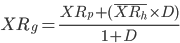The resulting value is then used to compute the Net XR value (XRn), which should never fall below 0.50.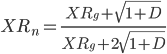Fig. G3: An XR = 0.93 Stemm and its Halo can be interpreted as a MetaStemm of XR = 0.58 by way of the Dark Matter solution. (Halo rings are speculative, values for example only.)

Thus, the Halo contributes to the overall mass of the Stemm in the form of an imperceptible mass/energy, even though the contribution is not directly measurable due to being comprised of iStemms.

RULE G6: In contrast to the MetaStemm, which is a group of Stemms interpreted as a single Stemm, any single Stemm (or MetaStemm) can be visualized as a Compound Stemm composed of any number of equal Stemms.Fig. G1: A XR=0.96 vStemm as a Compound Stemm. However, these component Stemms could be higher ratio Stemms that are mutually slowed to 0.96 by each other’s halos.

Since Stemms can carry more than one Stemm State, a vStemm State that occupies the Stemm of a Stemm Halo (or any iStemm) is influenced by the underlying iStemms. Since the Stemm State of a Halo Stemm is transitioned smoothly by way of interpretation as a Compound Stemm, the interceding vStemm State’s ratio is lowered (towards 1:1) on the side nearest the base stemm. This way, the intervening vStemm has become distorted and is drawn towards the base Stemm.

This is the mechanism behind gravity, which is a vStemm-iStemm interaction, unlike strong and electroweak forces that are governed by vStemm-vStemm interactions. This solves the Hierarchy Problem, by placing gravity as an effect of much smaller iStemms.

RULE G7: To determine how a vStemm is affected by an iStemm halo, use the tentative Percentage Solution. Multiply the ratio of the vStemm by the ratio of the underlying iStemms as a percentage via:In a field of 1:0 stemms, the XR value of the vStemm will remain the same (0.96 × 1 = 0.96). However, within a Stemm Halo, where the XR value transitions towards 1:0 away from the Parent stemm, the vStemm’s MetaStemm components’ XR values are lowered to the side nearest the Parent stemm as illustrated in Fig G2.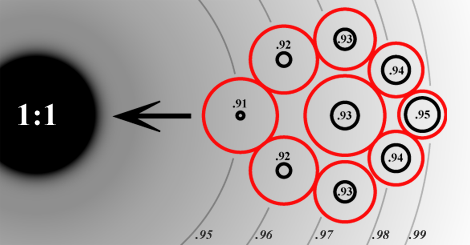Fig. G2: An XR=0.96 vStemm within the Stemm Halo of a 1:1 Stemm. The underlying halo iStemm States (grey lines) interact with the components of the Compound vStemm and are ‘smeared’ evenly. The energy differential causes the Compound Stemm to be drawn towards the 1:1 Stemm. The ratio of the vStemm is now lowered to 0.93 (by adding the various values and averaging). Not pictured is the Stemm Halo of the 0.96 Stemm and the equivalent, though greatly diminished effect it would have on the 1:1 Stemm. Values are exaggerated for emphasis.

RULE G8: This lowering of a vStemms overall ratio does not further increase that Stemm’s Halo, as this would lead to a runaway feedback loop whereby any two vStemms in each other’s Halo would reduce each other’s XR value to 1:1.

The effect of the Percentage Solution is only perceptible from outside the reference frame. Any object within the local reference frame would be similarly affected, and the 0.96 Stemm would appear retain its origional ratio. This increase in apparent mass (XR = 0.93 from 0.96) appears contrary to the concept of the Continuum based on velocity established via Rule B1. (but is in line with the Standard Model concept of Relative Mass) This paradoxical effect will be discussed below in I. Wave Effects and Off Sequence Stemms.

## H. Acceleration Effect vs. Affect

As seen above, vStemms are affected by the underlying iStemms through which they propagate. The amount of attraction they experience towards the parent Stemm is based not on absolute values, but on the slope of the Halo transitions. In Figure G2, the components of the MetaStemm correspond to five tiers of Stemm Halo, from left to right, 0.95, 0.96, 0.97, 0.98 and 0.99. (In reality, XR values of iStemms would never be lower than those of vStemms, but for illustrative purposes, these values are sufficient). This slope can be defined as 1:1, meaning that for every successive layer of the Halo, the XR value of the underlying Halo Stemm increases by one unit (which here 0.01 is given as an example; in reality the Halo would be defined by the limits set forth in Rule G1, G2 and G3).

However, at the same distance from a lower ratio Parent Stemm, or closer to the same vStemm, the slope would become steeper. For, example, if our vStemm moves to where the slope is 1:2, the underlying iStemms of the Stemm Halo might be 0.91, 0.93, 0.95, 0.97, and 0.99, giving our vStemm a new average of (XR = 0.91) This stemm is observed to be under greater acceleration, as the difference between XR values at opposite ends of the Stemm has increased.

In Stemm Theory, this phenomena is the cause of acceleration, and not a by-product of it. The perceived affects of it, such as gravity or g-forces from acceleration, are identical. Thus, any scenario where acceleration is involved, such as a satellite falling out of orbit, a spaceship launching, even the application of force such as moving a table across a floor, is inducing this kind of change in the Stemms that comprise the accelerating object.

The mechanism of this attraction in Stemm Theory is simply the process of equalization of all Stemms that are under co-influencing conditions. If the rate of propagation of a Stemm is such that it counteracts the equalization, the two Stemms are perceived to orbit each other. If the rate of propagation is less than the equalization, then the two Stemms will collide, or pass through each other, depending on their XR values in accordance with Rule F1.

All processes described through the Standard Model of physics can be interpreted as Stemm functions.

## I. Wave Effects and Off Sequence Stemms

Before addressing wave effects, the transparency of their origin needs to be stated. Consider some possible scenarios where the wavelength of light is long, but its velocity remains equal to that of light with a shorter wavelength.

1) A distant galaxy whose white light has been redshifted due to space-time expansion.

2) A massive, nearby star whose white light is redshifted by the gravity of the star.

3) A spaceship speeding away, emitting a white light that is redshifted by a doppler effect.

4) A nearby source emitting a red light.

In all these cases, without knowing the source of the light, the properties of the red light waves would all be indistinguishable from each other. Therefore the effect of the first three scenarios has on a Stemm is the same. In this sense, gravity is functionally equivalent to velocity away from the observer, as is the expansion of intervening space. For simplicity, only one need be described to establish a mechanism that will work for all three, and be indistinguishable from the fourth.

Following the example of how Stemms are warped by Stemm Halos as in Figure G2, above: if the illustrated vStemm were propagating towards the 1:1 Stemm, the Compound Stemm components on the leading edge would be traveling slower than those at the trailing edge. This causes the vStemm to compress parallel to the direction of travel, thus reducing the wavelength, creating blueshift. If the direction is reversed, away from the 1:1 Stemm, the leading edge propagates faster than the trailing edge, causing the vStemm to expand, creating redshift.

The increase in mass can be explained by the effective X value of the Stemm remaining the same (maintaining propagation velocity as defined by the Exponent Solution, Rule F4) which is in line with the Standard Model of relativistic mass, whereby acceleration causes mass to increase. Thus, the Redshifted Stemm is perceived to be Off Sequence.Fig. I1: A vStemm that has been affected by passing through an iStemm field becomes Off Sequence. The blue dotted line represents expected Main Sequence Characteristics according to Rule E5. Instead, the off sequence Stemm maintains the same X value.

The effect of an off sequence Stemm is that the wavelength has increased (due to the area where the vStemm exists increasing (Observable 'size' is Xe).

It has been observed that light wave effects can be simulated by a drop of oil on a vibrating surface, which is analogous to the expansion/contraction of Stemms, and further reinforces the core concept of Stemm Theory. In this way, as the vibrating Stemm is elongated or compressed, it creates the observed 2 dimensional waveform undergoing blue or redshift, respectively.

The exact same elongation and compression is created by The Metric Expansion of Space (the higher expansion rate of 1:0 iStemms, #1 above) and by a local Doppler Effect (#3 above)

## J. vStemm State Interactions

When two vStemm States come into contact (i.e. attempt to propagate into the same stemm), their interactions can be described on a higher level using Standard Model strong and electroweak interactions.

However, when allowed by Rule F1 established above, two Stemm States can occupy the same Stemm at the same time. Since this possibility outside of 1:1 Stemms is always nonzero, it also means that there is a minute chance that very massive MetaStemms can move through other massive MetaStemms.

When two stemm states interact and are not simultaneous, they are co-influenced by each others halos, combining into a higher mass MetaStemm.

 Actual XR 0.98 0.97 0.95 0.968 0.97 0.93 0.967 0.969 vStemms 0.95 0.968 0.97 0.93 0.967 0.969 0.95 0.968 Halo iStemms 0.99 0.97 0.95 <------------------ --------> 0.997 0.998 0.999 Observed XR 0.95 0.968 MetaStemm 0.959

Table J1: Two vStemms interact with each other’s Halo to create a MetaStemm of Lower Energy / Higher Mass. The effect the 0.97 Stemm has on the 0.98 Stemm is greater due to its higher mass. Additional vStemms would further decrease the MetaStemm’s XR value, creating very high mass MetaStemms. The actual XR can be further quantified with the Dark Matter Solution.

As other vStemms are added, due to Rule F1 that states that more than one Stemm State can occupy the same Stemm, the Multiple Halos add their influence on the vStemms, reducing the XR values of all the vStemms. This compounding effect is cumulative and can be approximated with the Averaging Solution:

RULE J1: The Ratio of a group of co-influencing vStemms (A MetaStemm, XRm) is simply equal to the Average Ratio of all vStemms.Using the Example above in Table J1, The Averaging Solution gives an XR for the MetaStemm of 0.96.

The averaging solution provides for a theoretical way to interpret a grouping of Stemms, however, the actual XR value would be observed to be lower due to the influence of Dark Matter using the Dark Matter Solution.

## K. Dark Matter and Dark Energy

Dark Matter is mass/energy associated with Stemm Halos, or all iStemms that that are greater than XR = 1:0 and where X < ℓP. Dark energy is ‘empty space’, or all iStemms that are not in a vStemm halo and at or near XR = 1:0.

The observed breakdown of Dark Energy, Dark Matter and visible Matter in the universe is 68.3%, 26.8% and 4.9% respectively. If this holds true across all scales, then Gravitational iStemm Halos would account for 26.8% of the universe, while 4.9% are vStemms, and the remaining 68.3% is 'empty' 1:0 iStemms.

RULE K1: Since Stemm Halos (as Dark Matter) comprise 26.8% of the mass-energy of the universe, Matter (Observable Stemms) is related to Dark matter in proportions defined by the Dark Matter Coefficient, which is simply the Universal ratio of vStemms to Halo iStemms: 26.8 / 4.9 or 1:5.47.

By Rule G5 and Figure G1 above, a MetaStemm was calculated to have XR = 0.58 using the Dark Matter Coefficient. However, by ignoring the Dark Matter Coefficient, the Stemm is observed to have XR = 0.96, making its physical perception much less massive on the mass/energy continuum.

As all groupings of Stemms can form a single Stemm via Compounds and Metas: Entire Galaxies can be interpreted as a single Stemm. Since the energy of Stemm Halo Stemms increase as the distance from the parent Stemm increases:

RULE K2:  the energy of a Stemm Halo becomes greater towards the fringes, not towards the core. This balances out the total Mass-Energy (in the form of vStemms at the center of the Halo), giving the observed universal density profiles of galaxies.

As gravity affects objects on galactic scales (e.g. pulling the Milky Way and Andromeda Galaxies together, and Galactic Clusters towards each other) Dark Matter as Gravity is certainly pervasive enough to constitute 26.8% of the mass-energy of the universe.

## L. Higgs and Other Particles

The existence of the Higgs particle is not precluded by the concepts of Stemm Theory, however, its function of giving mass to other particles is subsumed into the very nature of the Stemms themselves.

As Stemm Theory posits a continuum of Stemm States, an infinite number of different particles can theoretically exist as long as they do not violate any constraints. The Higgs particle would necessarily be interpreted as an iStemm, and its function in the Standard Model is not necessarily incompatible with Stemm Theory.

## M. Anti-Matter

As the universe is composed of Stemms that expand and contract at different ratios, for conventionally perceptible Stemms (elementary particles such as quarks), this expansion/contraction happens more or less simultaneously across the entire universe. All Stemms expand at the same time, then contract at the same time.

However, if the expansion/contraction phases are reversed, this creates anti-matter. When anti-matter contacts its corresponding matter (a Stemm of the exact same ratio, but reversed), the two expansion/contraction phases cancel each other out, and both the matter and antimatter are effectively annihilated.

The creation of the universe, in part, would have been enabled by the domination of one phase over the other, and may be linked to an “expansion first” big bang. e.g. For the universe to come into existence, the expansion of the first Stemm must have come before the contraction, and thus all subsequent stemms would be in sync with that.

## N. Suprasegmental Stemm Properties

In the standard theory of particle physics, each elementary particle has additional properties of spin, electric charge, and color charge.

Spin is an angular momentum that in Stemm Theory (contrary to the Standard Model which asserts that spin cannot be explained by breaking a particle down into smaller parts), spin is a suprasegmental property of a theoretical Compound Stemm, where internal energy fluctuations of increasing and decreasing off sequence stemms cause the Compound Stemm to exhibit rotation due to to the effects that the Off-Sequence stemms have. How far off sequence the fluctuations are along two-dimensional plane dictate the intensity of the spin, leading to commonly assigned values of ½, 1, etc.Fig. N1: A 7:3 Meta vStemm exhibits spin due to the off sequence Stemms that compose it. The average of all component Stemms averages to 7:3.

Since spin is non-negative, the direction of spin is irrelevant. Isospin could be explained with a similar mechanism along a different plane.

Charge could be derived from more complicated Suprasegmental phenomena, or could be derived from an out of sync expansion/contraction phase, identical or distinct from Anti-Matter.

## O. Time in Stemm Theory

### 1. Special Considerations

Since time is only observed to flow in one direction, it's unique properties must be interpreted differently from other dimensions.

In the Standard Model (and in Stemm Theory), an object traveling at c will be observed to experience no time. This is due to the finite nature of c, which is governed by the maximum possible rate of Stemm State propagation based on the Hubble Constant (Rule F5).

If there were no limits on c then events in the universe could happen simultaneously. Time therefore is a necessary by-product of the inability to exceed the limit of c, and not as an independent dimension in its own right.

To understand the unidirectionality of time, consider a thought experiment.

If object X observes object Y to be experiencing time, then object Y must be traveling at less than c from X's perspective. However, from the perspective of a 3rd object Z, both X and Y must both be traveling roughly in the same direction with respect to c.

Were X and Y traveling in opposite directions, and their combined speed was greater than c from Z's perspective, then X would necessarily observe Y to be traveling at c, and vice versa. Neither X nor Y would observe the other to be experiencing time.

In the frame of X and Y, if X were stationary and observing that Y is experiencing no time, it would mean that Y was moving either away or towards X at c. In order for X to observe Y experiencing time, X must move in the same direction as Y, in order to subtract from Y's observed speed of c.

Thus, in a very literal sense, Time only exists in one direction.

### 2. Uncertainty and Relevance

The uncertainty of time in Stemm Theory arises from a principle similar to Heisenberg Uncertainty. Since Time in Stemm Theory is understood to be a byproduct of the limit of c, it is also analogous to measurements of distance or size in that all of these measurable quantities become increasingly uncertain as their values decrease.

This uncertainty can be interpreted as whether or not time is relevant.

RULE O1: As Stemm ratio decreases towards 1:0, time does not slow down as much as the need for time becomes less relevant, since any observed change of the Stemm (via propagation or interaction) becomes less certain.

Using the extremely tentative Time Solution, a Stemm’s contraction value (Xc) can be divided from that of the observer (Xco) to generate a percentage of time (tR).For example for a MetaStemm of 100:99 observing a vStemm of 7:3, the percentage of time observed is 1 ÷ (99 ÷ 3) × 100 = 3.03, or the vStemm experiences 3.03% of the time of the MetaStemm.

# III. The Way Forward

Any further work on Stemm Theory would require a higher level of scrutiny to the rules already established, including:

1) The various solutions presented need to be approached more rigorously. Particularly the Dark Matter solution, which generates lower XR values than expected.

2) Tighten the limits for Halo Stemms (Rules G1-G3) and define an equation that would fit within those limits.

3) attempt to define some real X and XR values for various known particles, and compute their actual Halo Values to see if the Percentage Solution (G7) is appropriate to describe how iStemms interact with vStemms, and by extension, how vStemms interact with each other.

4) investigate the assumption that all Stemms in the universe expand and contract in sync. This is only problematic in its unlikelihood, and would require a rethinking of anti-matter, but it could easily solve the question of the origin of Electric Charge.

5) Refine the Time Solution (O1) to equate to the observed effects already derived by the Lorentz Equation.

# IV. Appendix

## A. Rules

RULE B1: The only difference between Mass and Energy is velocity.

RULE D1: Even seemingly insignificant changes in velocity are extremely important.

RULE D2: Because of the asymptotic nature of c in relation to the Lorentz Factor, there are an infinite number of universes that have their own relative differentials between a stationary object, and one that is traveling at c, which are parallel to this universe.

RULE E1: For the average of all stemms, contraction is less than expansion, Xc < Xe.

RULE E2: For a stem to be “visible”, X must exceed or meet the Planck Length, Xe >= ℓP.

RULE E3: The measurable size of all stemms is equal to Xe.

RULE E4: As net expansion of any Stemm can only be measured over great distances or time: no Stemm has an X value greater than the Planck Length.

RULE E5: Stemms can be plotted along a Main Sequence where the expansion size and the ratio are inversely proportional to each other.

RULE E6: after the contraction phase is complete, the initial stemm has now expanded to a greater size, but maintains the same ratio value XR.

RULE E7: Over time, the expansion of all other Stemms approaches 0 compared to the expansion of ‘empty space’ 1:0 iStemms.

RULE F1: More than one Stemm State can occupy the same Stemm. As the XR ratio of a Stemm State decreases from 1:0, it’s ability to be simultaneous with another Stemm State rapidly decreases from 100%.

RULE F2: c can be stated as a velocity relative to the expansion of spacetime (1:0 Stemms):RULE F3: All matter is composed of vStemms that have XR values very near 1:0.

RULE F4: Use the Exponent Solution to calculate velocity relative to the Hubble Constant (vR) by solving for the X value of the vStemm (Xv) to the power of the X value of the iStemm (Xi):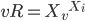RULE G1: Halo Stemms must never exceed the Planck Length (they are always iStemms). The X value of the innermost Halo Stemm of a 1:1 Parent is at or near the Planck Length.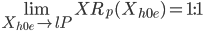RULE G2: As distance from the Parent Stemm increases, the XR value of the Stemm Halo meets and blends into the ‘empty space’ background of 1:0 iStemms..

RULE G3: As the Parent Stemm’s XR value approaches 1:0, the XR values of the Parent Stemm and the innermost Halo Stemm converge:RULE G4: As the Parent Stemm and the Halo become less distinct, the parent can only be quantified with less and less certainty.

RULE G5: Parent Stemms plus their Stemm Halos can be interpreted as a single Meta Stemm, whose ratio is derived with the tentative Dark Matter Solution:RULE G6: Any single Stemm (or MetaStemm) can be visualized as a Compound Stemm composed of any number of equal Stemms.

RULE G7: By the Percentage Solution for effects of Stemm Halos, multiply the ratio of the vStemm by the ratio of the underlying iStemms as a percentage via: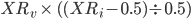RULE G8: This lowering of a vStemms overall ratio does not further increase that Stemm’s Halo.

RULE J1: The Ratio of a group of co-influencing vStemms (A MetaStemm, XRm) is equal to the Average Ratio of all vStemms.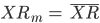RULE K1: The Dark Matter Coefficient, is simply the Universal ratio of vStemms to Halo iStemms: 26.8 / 4.9 gives a value of 5.47.

RULE K2:  the energy of a Stemm Halo becomes greater towards the fringes, not towards the core.

RULE O1: As Stemm ratio decreases towards 1:0, time does not slow down as much as the need for time becomes less relevant.

## B. Solutions

The Exponent Solution: By positing that vStemm states Propagate at speeds as a percentage of the relation to the Hubble Constant, then for a theoretical vStemm that travels at c, its velocity must be 18.60878 orders of magnitude more than the expansion rate of a 1:0 Stemm.

The exponent solution is to calculate the relative velocity (vR) by solving for the X value of the vStemm (Xv) to the power of the X value of the iStemm (Xi):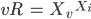The Dark Matter Solution: XR values for Parent Stemms plus their Stemm Halos can be derived using the Dark Matter Coefficient.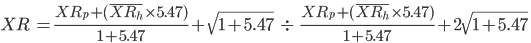The Percentage Solution: In order to calculate the effects of Simultaneous iStemms on vStemms: multiply the ratio of the vStemm by the ratio of the underlying iStemms as a percentage.The Averaging Solution: The Ratio of a group of co-influencing vStemms is equal to the Average Ratio of all vStemms.The Time Solution: A Stemm’s contraction value (Xc) can be divided from that of the observer (Xco) to generate a percentage of time observed (tR).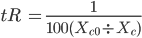The idea of universal simultaneity has been put forth before, unsuccessfully. Stemm theory only requires that the perception be simultaneous. Two events that take place within Planck Time are by perception simultaneous.

 Couder, Fort, et al. http://www.youtube.com/watch?v=W9yWv5dqSKk